# 5.4.5 - Factor Analysis - RESULTS - Reports - Teradata Warehouse Miner

## Teradata Warehouse Miner User Guide - Volume 3Analytic Functions

Product
Release Number
5.4.5
Published
February 2018
Language
English (United States)
Last Update
2018-05-04
dita:mapPath
yuy1504291362546.ditamap
dita:ditavalPath
ft:empty
dita:id
tbj1499730075274
1. On the Factor Analysis dialog box, click RESULTS.
2. Click reports.
The RESULTS tab is grayed-out/disabled until after the analysis is completed.
Factor Analysis > Results > Reports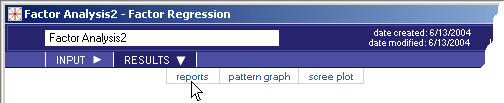## Data Quality Reports

• Variable Statistics — If selected on the Results Options tab, this report gives the mean value and standard deviation of each variable in the model based on the SSCP matrix provided as input.
• Near Dependency — If selected on the Results Options tab, this report lists collinear variables or near dependencies in the data based on the SSCP matrix provided as input. Entries in the Near Dependency report are triggered by two conditions occurring simultaneously. The first is the occurrence of a large condition index value associated with a specially constructed principal factor. If a factor has a condition index greater than the parameter specified on the Results Option tab, it is a candidate for the Near Dependency report. The other is when two or more variables have a variance proportion greater than a threshold value for a factor with a high condition index. Another way of saying this is that a ‘suspect’ factor accounts for a high proportion of the variance of two or more variables. The parameter to defines what a high proportion of variance is also set on the Results Option tab. A default value of 0.5.
• Detailed Collinearity Diagnostics — If selected on the Results Options tab, this report provides the details behind the Near Dependency report, consisting of the following tables.
• Eigenvalues of Unit Scaled X'X — Report of the eigenvalues of all variables scaled so that each variable adds up to 1 when summed over all the observations or rows. In order to calculate the singular values of X (the rows of X are the observations), the mathematically equivalent square root of the eigenvalues of XTX are computed instead for practical reasons
• Condition Indices — The condition index of each eigenvalue, calculated as the square root of the ratio of the largest eigenvalue to the given eigenvalue, a value always 1 or greater.
• Variance Proportions — The variance decomposition of these eigenvalues is computed using the eigenvalues together with the eigenvectors associated with them. The result is a matrix giving, for each variable, the proportion of variance associated with each eigenvalue.

## Principal Component Analysis Report

• Number of Variables — This is the number of variables to be factored, taken from the matrix that is input to the algorithm. Note that there are no dependent or independent variables in a factor analysis model.
• Minimum Eigenvalue — The minimum value of a factor’s associated eigenvalue, determining whether or not to include the factor in the final model. This field is not displayed if the Number of Factors option is used to determine the number of factors retained.
• Number of Factors — This value reflects the number of factors retained in the final factor analysis model. If the Number of Factors option is explicitly set by the user to determine the number of factors, then this reported value reflects the value set by the user. Otherwise, it reflects the number of factors resulting from applying the Minimum Eigenvalue option.
• Matrix Type (cor/cov) — This value reflects the type of input matrix requested by the user, either correlation (cor) or covariance (cov).
• Rotation (none/orthogonal/oblique) — This value reflects the type of rotation, if any, requested by the user, either none, orthogonal, or oblique.
• Gamma — This value is a coefficient in the rotation equation that reflects the type of rotation requested, if any, and in some cases is explicitly set by the user. Gamma is determined as follows.
• Orthogonal rotations
• Varimax — (gamma in rotation equation fixed at 1.0)
• Quartimax — (gamma in rotation equation fixed at 0.0)
• Equamax — (gamma in rotation equation fixed at f / 2)*
• Parsimax — (gamma in rotation equation fixed at v(f-1) / (v+f+2))*
• Orthomax — (gamma in rotation equation set by user)

* where v is the number of variables and f is the number of factors

• Oblique rotations
• Quartimin — (gamma in rotation equation fixed at 0.0)
• Biquartimin — (gamma in rotation equation fixed at 0.5)
• Covarimin — (gamma in rotation equation fixed at 1.0)
• Orthomin — (gamma in rotation equation set by user)

## Principal Axis Factors Report

• Number of Variables — Number of variables to be factored, taken from the matrix that is input to the algorithm. Note that there are no dependent or independent variables in a factor analysis model.
• Minimum Eigenvalue — Minimum value of a factor’s associated eigenvalue, determining whether or not to include the factor in the final model. This field is not displayed if the Number of Factors option is used to determine the number of factors retained.
• Number of Factors — Number of factors retained in the final factor analysis model. If the Number of Factors option is explicitly set by the user to determine the number of factors, then this reported value reflects the value set by the user. Otherwise, it reflects the number of factors resulting from applying the Minimum Eigenvalue option.
• Maximum Iterations — This is the maximum number of iterations requested by the user.
• Convergence Criterion — Value requested by the user as the convergence criterion such that iteration continues until the maximum change in the square root of uniqueness values does not exceed this value.
• Rotation (none/orthogonal/oblique) — Type of rotation, if any, requested by the user, either none, orthogonal, or oblique.
• Gamma — Value is a coefficient in the rotation equation that reflects the type of rotation requested, if any, and in some cases is explicitly set by the user. Gamma is determined as follows.
• Orthogonal rotations
• Varimax — (gamma in rotation equation fixed at 1.0)
• Quartimax — (gamma in rotation equation fixed at 0.0)
• Equamax — (gamma in rotation equation fixed at f / 2)*
• Parsimax — (gamma in rotation equation fixed at v(f-1) / (v+f+2))*
• Orthomax — (gamma in rotation equation set by user)

* where v is the number of variables and f is the number of factors

• Oblique rotations
• Quartimin — (gamma in rotation equation fixed at 0.0)
• Biquartimin — (gamma in rotation equation fixed at 0.5)
• Covarimin — (gamma in rotation equation fixed at 1.0)
• Orthomin — (gamma in rotation equation set by user)

## Maximum Likelihood (EM) Factor Analysis Report

• Number of Variables — Number of variables to be factored, taken from the matrix that is input to the algorithm. Note that there are no dependent or independent variables in a factor analysis model.
• Number of Observations — Number of observations in the data used to build the matrix that is input to the algorithm.
• Number of Factors — Number of factors requested by the user for the factor analysis model.
• Maximum Iterations — Maximum number of iterations requested by the user. The actual number of iterations used is reflected in the Total Number of Iterations field further down in the report.
• Convergence Criterion — Value requested by the user as the convergence criterion such that iteration continues until the maximum change in the square root of uniqueness values does not exceed this value.
Convergence is based on uniqueness values rather than maximum likelihood values, something that is done strictly for practical reasons based on experimentation.
• Matrix Type (cor/cov) — Type of input matrix requested by the user, either correlation (cor) or covariance (cov).
• Rotation (none/orthogonal/oblique) — Type of rotation, if any, requested by the user, either none, orthogonal, or oblique.
• Gamma — Value is a coefficient in the rotation equation that reflects the type of rotation requested, if any, and in some cases is explicitly set by the user. Gamma is determined as follows.
• Orthogonal rotations
• Varimax — (gamma in rotation equation fixed at 1.0)
• Quartimax — (gamma in rotation equation fixed at 0.0)
• Equamax — (gamma in rotation equation fixed at f / 2)*
• Parsimax — (gamma in rotation equation fixed at v(f-1) / (v+f+2))*
• Orthomax — (gamma in rotation equation set by user)

* where v is the number of variables and f is the number of factors

• Oblique rotations
• Quartimin — (gamma in rotation equation fixed at 0.0)
• Biquartimin — (gamma in rotation equation fixed at 0.5)
• Covarimin — (gamma in rotation equation fixed at 1.0)
• Orthomin — (gamma in rotation equation set by user)
• Total Number of Iterations — Number of iterations that the algorithm performed to converge on a maximum likelihood solution.
• Final Average Likelihood — Final value of the average likelihood over all the observations represented in the input matrix.
• Change in Avg Likelihood — Final change, from the previous to the final iteration, in value of the average likelihood over all the observations represented in the input matrix.
• Maximum Change in Sqrt (uniqueness) — Algorithm calculates a uniqueness value for each factor each time it iterates, and keeps track of how much the positive square root of each of these values changes from one iteration to the next. The maximum change in this value is given here, and it is of interest because it is used to determine convergence of the model. Refer to Final Uniqueness Values later in this section for an explanation of these values in the common factor model.

## Max Change in Sqrt (Communality) For Each Iteration

This report, printed for Principal Axis Factors only, and only if the user requests the Report Output option Long, shows the progress of the algorithm in converging on a solution. It does this by showing, at each iteration, the maximum change in the positive square root of the communality of each of the variables. The communality of a variable is that portion of its variance that can be attributed to the common factors. Simply put, when the communality values for all of the variables stop changing sufficiently, the algorithm stops.

## Matrix to be Factored

The correlation or covariance matrix to be factored is printed out only if the user requests the Report Output option Long. Only the lower triangular portion of this symmetric matrix is reported and output is limited to at most 100 rows for expediency.

If it is necessary to view the entire matrix, the Get Matrix function with the Export to File option is recommended.

## Initial Communality Estimates

This report is produced only for Principal Axis Factors and Maximum Likelihood Factors. The communality of a variable is that portion of its variance that can be attributed to the common factors, excluding uniqueness. The initial communality estimates for each variable are made by calculating the squared multiple correlation coefficient of each variable with respect to the other variables taken together.

## Final Communality Estimates

This report is produced only for Principal Axis Factors and Maximum Likelihood Factors. The communality of a variable is that portion of its variance that can be attributed to the common factors, excluding uniqueness. The final communality estimates for each variable are computed as: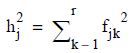(i.e., as the sum of the squares of the factor loadings for each variable).

## Eigenvalues

These are the resulting eigenvalues of the principal component or principal axis factor solution, in descending order. At this stage, there are as many eigenvalues as input variables since the number of factors has not been reduced yet.

## Eigenvectors

These are the resulting eigenvectors of the principal components or principal axis factor solution, in descending order. At this stage, there are as many eigenvectors as input variables since the number of factors has not been reduced yet. Eigenvectors are printed out only if the user requests the Report Output option Long.

This matrix of values, which is variables by factors in size, represents both the factor pattern and factor structure, i.e., the linear combination of factors for each variable and the correlations between factors and variables (provided Matrix Type is Correlation). The number of factors has been reduced to meet the minimum eigenvalue or number of factors requested, but the output does not reflect any factor rotations that may have been requested.

This output table contains the raw data used in the Prime Factor Reports, which are probably better to use for interpreting results. If the user requested a Matrix Type of Correlation, the principal component loadings can be interpreted as the correlations between the original variables and the newly created factors. An absolute value approaching 1 indicates that a variable is contributing strongly to a particular factor.

## Factor Pattern (Principal Axis Factors)

This matrix of values, which is variables by factors in size, represents both the factor pattern and factor structure, i.e., the linear combination of factors for each variable and the correlations between factors and variables (provided Matrix Type is Correlation). The number of factors has been reduced to meet the minimum eigenvalue or number of factors requested, but the output does not reflect any factor rotations that may have been requested.

This output table contains the raw data used in the Prime Factor Reports, which are probably better to use for interpreting results. If the user requested a Matrix Type of Correlation, the factor pattern can be interpreted as the correlations between the original variables and the newly created factors. An absolute value approaching 1 indicates that a variable is contributing strongly to a particular factor.

## Factor Pattern (Maximum Likelihood Factors)

This matrix of values, which is variables by factors in size, represents both the factor pattern and factor structure, i.e., the linear combination of factors for each variable and the correlations between factors and variables (provided Matrix Type is Correlation). The number of factors has been fixed at the number of factors requested. The output at this stage does not reflect any factor rotations that may have been requested.

This output table contains the raw data used in the Prime Factor Reports, which are probably better to use for interpreting results. If the user requested a Matrix Type of Correlation, the factor pattern can be interpreted as the correlations between the original variables and the newly created factors. An absolute value approaching 1 indicates that a variable is contributing strongly to a particular factor.

## Variance Explained by Factors

This report provides the amount of variance in all of the original variables taken together that is accounted for by each factor. For Principal Components and Principal Axis Factor solutions, the variance is the same as the eigenvalues calculated for the solution. In general however, and for Maximum Likelihood Factor solutions in particular, the variance is the sum of the squared loadings for each factor.

After an oblique rotation, if the factors are correlated, there is an interaction term that must also be added in based on the loadings and the correlations between factors. A separate report entitled Contributions of Rotated Factors To Variance is provided if an oblique rotation is performed.
• Factor Variance — This column shows the actual amount of variance in the original variables accounted for by each factor.
• Percent of Total Variance — This column shows the percentage of the total variance in the original variables accounted for by each factor.
• Cumulative Percent — This column shows the cumulative percentage of the total variance in the original variables accounted for by Factor 1 through each subsequent factor in turn.

## Factor Variance to Total Variance Ratio

This is simply the ratio of the variance explained by all the factors to the total variance in the original data.

## Condition Indices of Components

The condition index of a principal component or principal factor is the square root of the ratio of the largest eigenvalue to the eigenvalue associated with that component or factor.

This report is provided for Principal Components and Principal Axis Factors only.

## Final Uniqueness Values

The common factor model seeks to find a factor pattern C and a uniqueness matrix R such that a covariance or correlation matrix S can be modeled as S = CCT + R. The uniqueness matrix is a diagonal matrix, so there is a single uniqueness value for each variable in the model. The theory behind the uniqueness value of a variable is that the variance of each variable can be expressed as the sum of its communality and uniqueness, that is the variance of the jth variable is given by: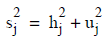This report is provided for Maximum Likelihood Factors only.

The results of a factor analysis can be used to reproduce or approximate the original correlation or covariance matrix used to build the factor analysis model. This is done to evaluate the effectiveness of the model in accounting for the variance in the original data. For Principal Components and Principal Axis Factors the reproduced matrix is simply the loadings matrix times its transpose. For Maximum Likelihood Factors it is the loadings matrix times its transpose plus the uniqueness matrix.

This report is provided only when Long is selected as the Output Option.

## Difference Between Original and Reproduced cor/cov Matrix

This report gives the differences between the original correlation or covariance matrix values used in the factor analysis and the Reproduced Matrix Based on Loadings. (In the case of Principal Axis Factors, the reproduced matrix is compared to the original matrix with the initial communality estimates placed in the diagonal of the matrix).

This report is provided only when Long is selected as the Output Option.

## Absolute Difference

This report summarizes the absolute value of the differences between the original correlation or covariance matrix values used in the factor analysis and the Reproduced Matrix Based on Loadings.
• Mean — Average absolute difference in correlation or covariance over the entire matrix.
• Standard Deviation — Standard deviation of the absolute differences in correlation or covariance over the entire matrix.
• Minimum — Minimum absolute difference in correlation or covariance over the entire matrix.
• Maximum — Maximum absolute difference in correlation or covariance over the entire matrix.

This report of the factor loadings (pattern) after rotation is given only after orthogonal rotations.

## Rotated Structure

This report of the factor structure after rotation is given only after oblique rotations. Note that after an oblique rotation the rotated structure matrix is usually different from the rotated pattern matrix.

## Rotated Pattern

This report of the factor pattern after rotation is given after both orthogonal and oblique rotations. Note that after an oblique rotation the rotated pattern matrix is usually different from the rotated structure matrix.

## Rotation Matrix

After rotating the factor pattern matrix P to get the rotated matrix PR, the rotation matrix T is also produced such that PR = PT. However, after an oblique rotation the rotation matrix obeys the following equation: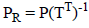.

This report is provided only when Long is selected as the Output Option.

## Variance Explained by Rotated Factors

This is the same report as Variance Explained by Factors except that it is based on the rotated factor loadings. Comparison of the two reports can show the effects of rotation on the effectiveness of the model.

After an oblique rotation, another report is produced called the Contributions of Rotated Factors to Variance to show both the contributions of individual factors and the contributions of factor interactions to the explanation of the variance in the original variables analyzed.

## Rotated Factor Variance to Total Variance Ratio

This is the same report as Factor Variance to Total Variance Ratio except that it is based on the rotated factor loadings. Comparison of the two reports can show the effects of rotation on the effectiveness of the model.

## Correlations Among Rotated Factors

After an oblique rotation the factors are generally no longer orthogonal or uncorrelated with each other. This report is a standard Pearson product-moment correlation matrix treating the rotated factors as new variables. Values range from 0 to -1 or +1 indicating no correlation to maximum correlation respectively (a negative correlation indicates that two factors vary in opposite directions with respect to each other).

This report is provided only after an oblique rotation is performed.

## Contributions of Rotated Factors to Variance

In general, the variance of the original variables explained by a factor is the sum of the squared loadings for the factor. But after an oblique rotation the factors may be correlated, so additional interaction terms between the factors must be considered in computing the explained variance reported in the Variance Explained by Rotated Factors report.

The contributions of factors to variance may be characterized as direct contributions: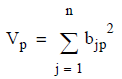and joint contributions: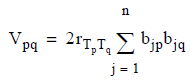where the following is true:
• p and q vary by factors with p < q
• j varies by variables
• r is the correlation between factors
The Contributions of Rotated Factors to Variance report displays direct contributions along the diagonal and joint contributions off the diagonal.

This report is provided only after an oblique rotation is performed.